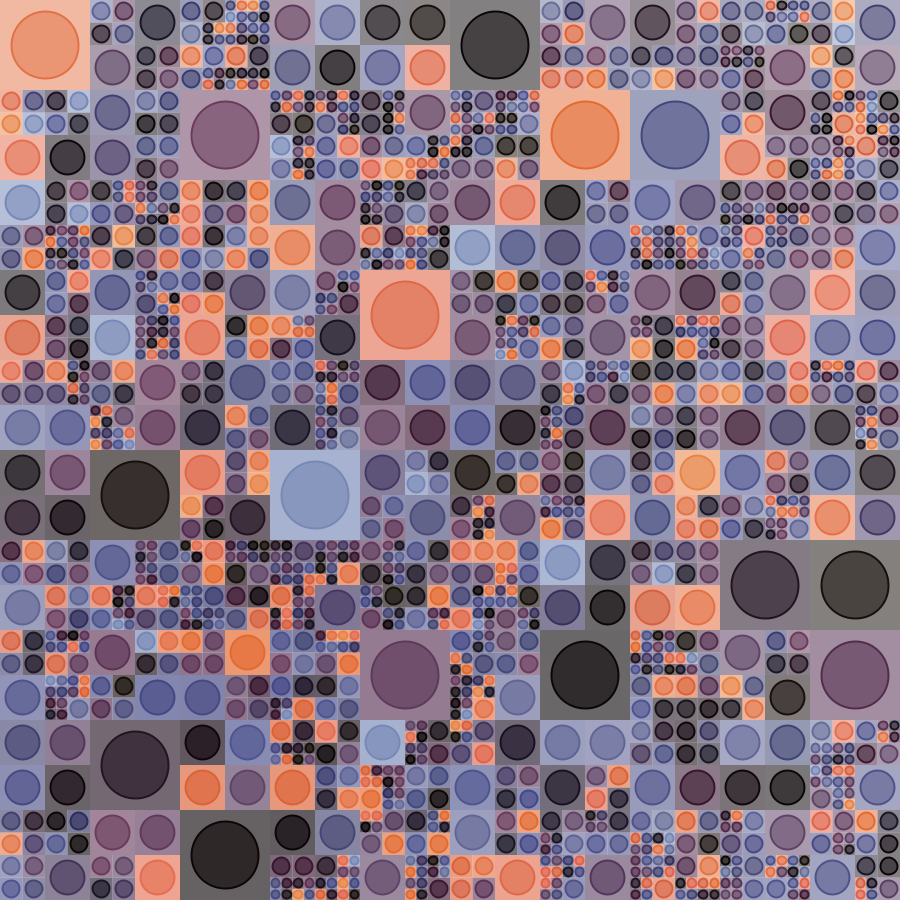## Grid based generative art (part 3) - colors from an image.

The following are the previous tutorials in this series:

In this tutorial, you will see how you can pick the colors for a grid cell based on the colors in any image of your choice. This can be done in a couple of different ways:

• For a grid cell, you choose a color based on the position of the cell and the color present at the corresponding position in the reference image.
• You create a palette out of the most frequently used colors in an image, and then you randomly choose from this palette (using a uniform or weighted strategy as described in the previous article).

### Approach 1 - Colors from the corresponding position in an image

The key idea is shown in the following code:

``````val img = image("some-image.png")
def imgColor(x: Double, y: Double) = {
val nx = mathx.map(x, 0, cwidth, 0, img.getWidth).toInt
val ny = mathx.map(y, 0, cheight, 0, img.getHeight).toInt
getImagePixel(img, nx, ny)
}
``````

For any grid position, the color from the corresponding position in the image can be obtained via the `imgColor` function defined above. This function makes use of `mathx.map(value, low1, high1, low2, high2)` to do its work. `mathx.map` maps the given value from the range (low1, high1) to the range (low2, high2).

Let’s see this in action with a complete program. The program will make an irregular grid of rectangles, and color it using the colors from an image.

Here’s the image: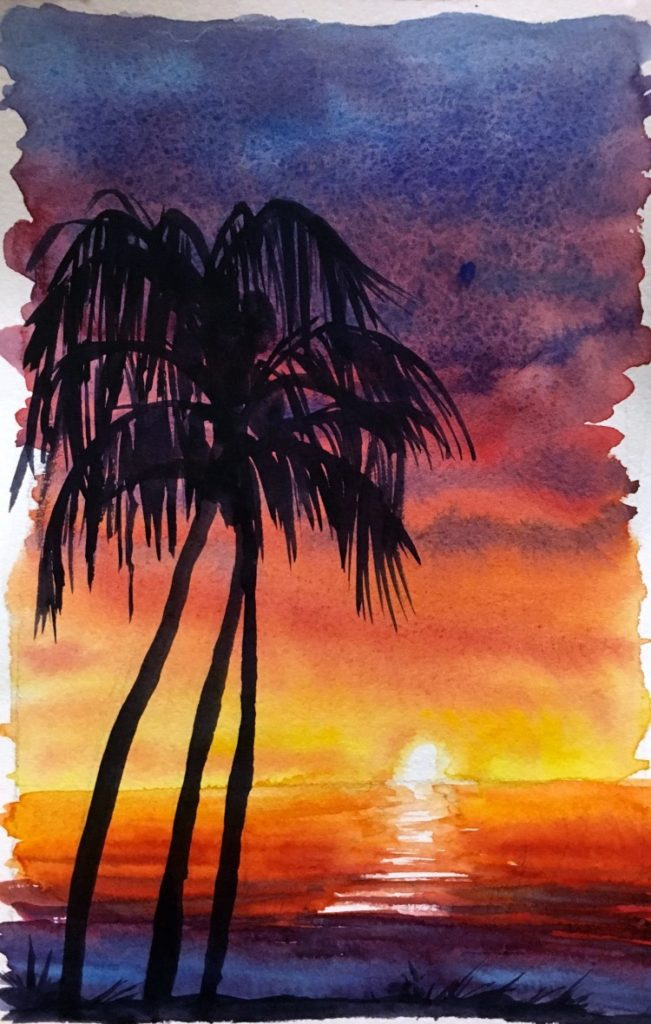And here’s the program that uses the colors from the image:

``````size(900, 900)
cleari()
setBackground(cm.white)
originBottomLeft()
initRandomGenerator(-8312538470690622046L)

val tileCount = 3
val tileWidth = cwidth / tileCount
val tileHeight = cheight / tileCount

// Todo - save the above image as sunset-tree.png and then use it in your program.
// without the above step, the program will not work.
val img = image("sunset-tree.png")
def imgColor(x: Double, y: Double) = {
val nx = mathx.map(x, 0, cwidth, 0, img.getWidth).toInt
val ny = mathx.map(y, 0, cheight, 0, img.getHeight).toInt
getImagePixel(img, nx, ny)
}

def shape(w: Double, h: Double) = {
Picture.rectangle(w, h)
}

case class Block(x: Double, y: Double, w: Double, h: Double) {
val c = imgColor(x, y)
}
var blocks = ArrayBuffer.empty[Block]

def drawBlock(b: Block) {
val pic = shape(b.w, b.h)
pic.setPosition(b.x, b.y)
val d = mathx.distance(b.x, b.y, mouseX, mouseY)
val f = mathx.map(d, 0, 800, 0.3, .9)
val angle = mathx.angle(b.x, b.y, mouseX, mouseY)
pic.setPenThickness(.5)
draw(pic)
}

def splitSomeBlocks(blocks: ArrayBuffer[Block], p: Double): ArrayBuffer[Block] = {
val blocks2 = ArrayBuffer.empty[Block]
repeatFor(blocks) { b =>
if (randomDouble(1) < p) {
val newBlocks = Array(
Block(b.x, b.y, b.w / 2, b.h / 2),
Block(b.x, b.y + b.h / 2, b.w / 2, b.h / 2),
Block(b.x + b.w / 2, b.y, b.w / 2, b.h / 2),
Block(b.x + b.w / 2, b.y + b.h / 2, b.w / 2, b.h / 2)
)
blocks2.appendAll(newBlocks)
}
else {
blocks2.append(b)
}
}
blocks2
}

setup {
repeatFor(rangeTill(0, cheight, tileHeight)) { posY =>
repeatFor(rangeTill(0, cwidth, tileWidth)) { posX =>
val block = Block(posX, posY, tileWidth, tileHeight)
blocks.append(block)
}
}
repeat(9) {
blocks = splitSomeBlocks(blocks, 0.7)
}
}

setup {
erasePictures()
repeatFor(blocks) { b =>
drawBlock(b)
}
}
``````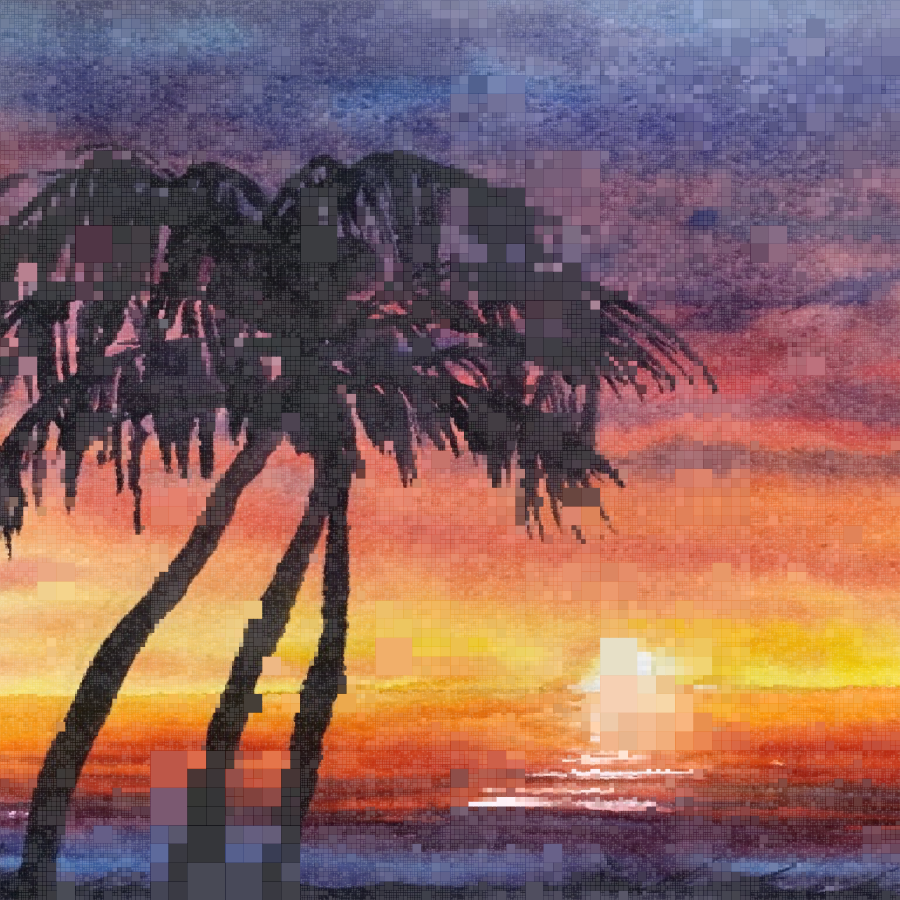Let’s try something similar with another image: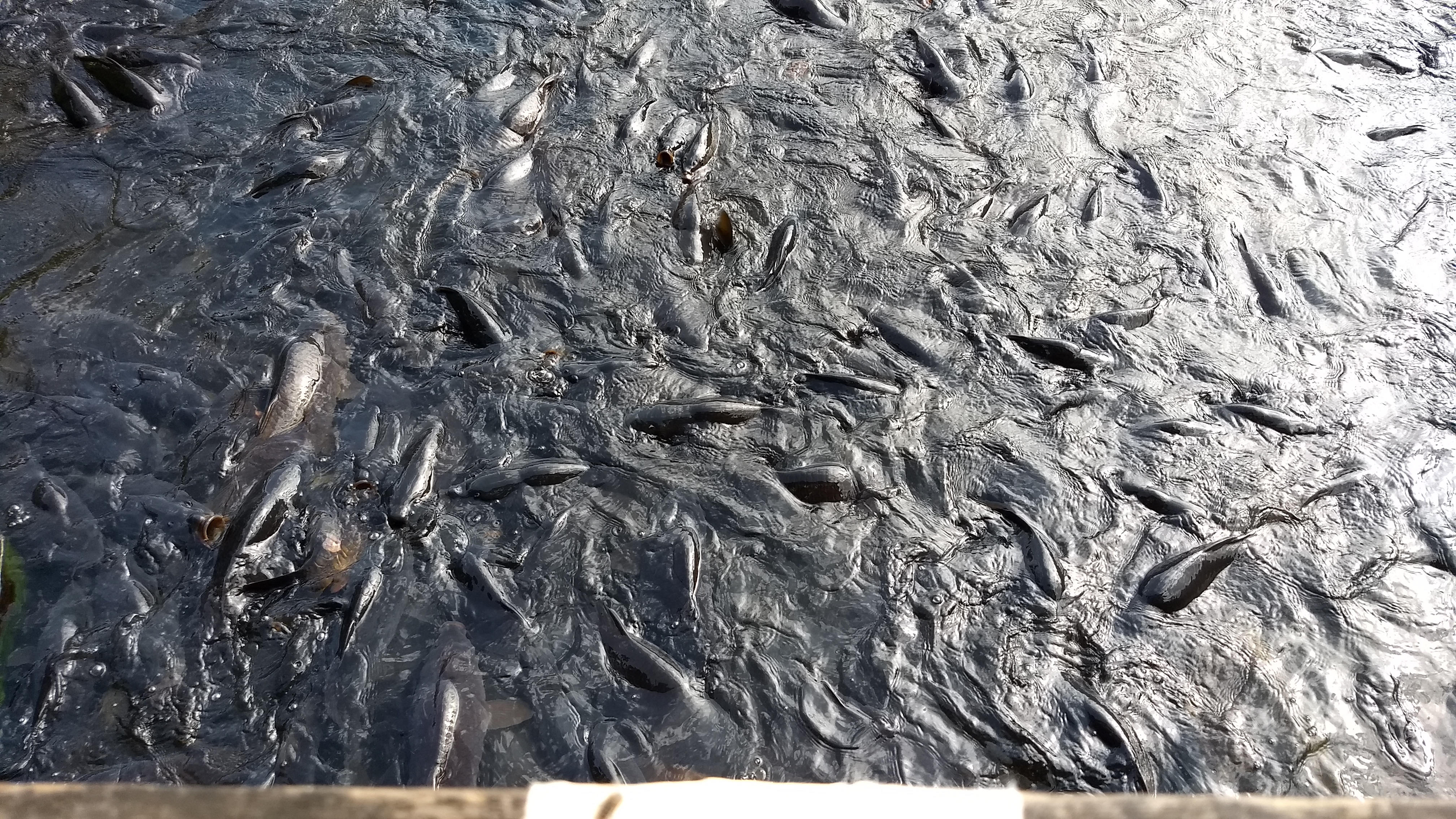Here’s the program that uses the colors from the image:

``````size(900, 900)
cleari()
setBackground(white)
originBottomLeft()
initRandomGenerator(-8563875002700992940L)

val tileCount = 5
val tileWidth = cwidth / tileCount
val tileHeight = cheight / tileCount

val img = image(url("http://docs.kogics.net/tutorials/gray-fish.png"))
def imgColor(x: Double, y: Double) = {
val nx = mathx.map(x, 0, cwidth, 0, img.getWidth).toInt
val ny = mathx.map(y, 0, cheight, 0, img.getHeight).toInt
getImagePixel(img, nx, ny)
}

def cellColor = randomColor

def shape(w: Double, h: Double) = {
val len = math.min(w, h) * 3 / 4
picStackCentered(noPen -> Picture.rectangle(w, h), Picture.ellipseInRect(len, len))
}

case class Block(x: Double, y: Double, w: Double, h: Double) {
val c = imgColor(x, y)
}
var blocks = ArrayBuffer.empty[Block]

def drawBlock(b: Block) {
val pic = shape(b.w, b.h)
pic.setPosition(b.x, b.y)
val d = mathx.distance(b.x, b.y, mouseX, mouseY)
val f = mathx.map(d, 0, 800, 0.3, .9)
val angle = mathx.angle(b.x, b.y, mouseX, mouseY)
pic.setPenColor(b.c)
pic.setPenThickness(2)
draw(pic)
}

def splitSomeBlocks(blocks: ArrayBuffer[Block], p: Double): ArrayBuffer[Block] = {
val blocks2 = ArrayBuffer.empty[Block]
repeatFor(blocks) { b =>
if (randomDouble(1) < p) {
val newBlocks = Array(
Block(b.x, b.y, b.w / 2, b.h / 2),
Block(b.x, b.y + b.h / 2, b.w / 2, b.h / 2),
Block(b.x + b.w / 2, b.y, b.w / 2, b.h / 2),
Block(b.x + b.w / 2, b.y + b.h / 2, b.w / 2, b.h / 2)
)
blocks2.appendAll(newBlocks)
}
else {
blocks2.append(b)
}
}
blocks2
}

setup {
repeatFor(rangeTill(0, cheight, tileHeight)) { posY =>
repeatFor(rangeTill(0, cwidth, tileWidth)) { posX =>
val block = Block(posX, posY, tileWidth, tileHeight)
blocks.append(block)
}
}
repeat(7) {
blocks = splitSomeBlocks(blocks, 0.5)
}
}

setup {
erasePictures()
repeatFor(blocks) { b =>
drawBlock(b)
}
}
``````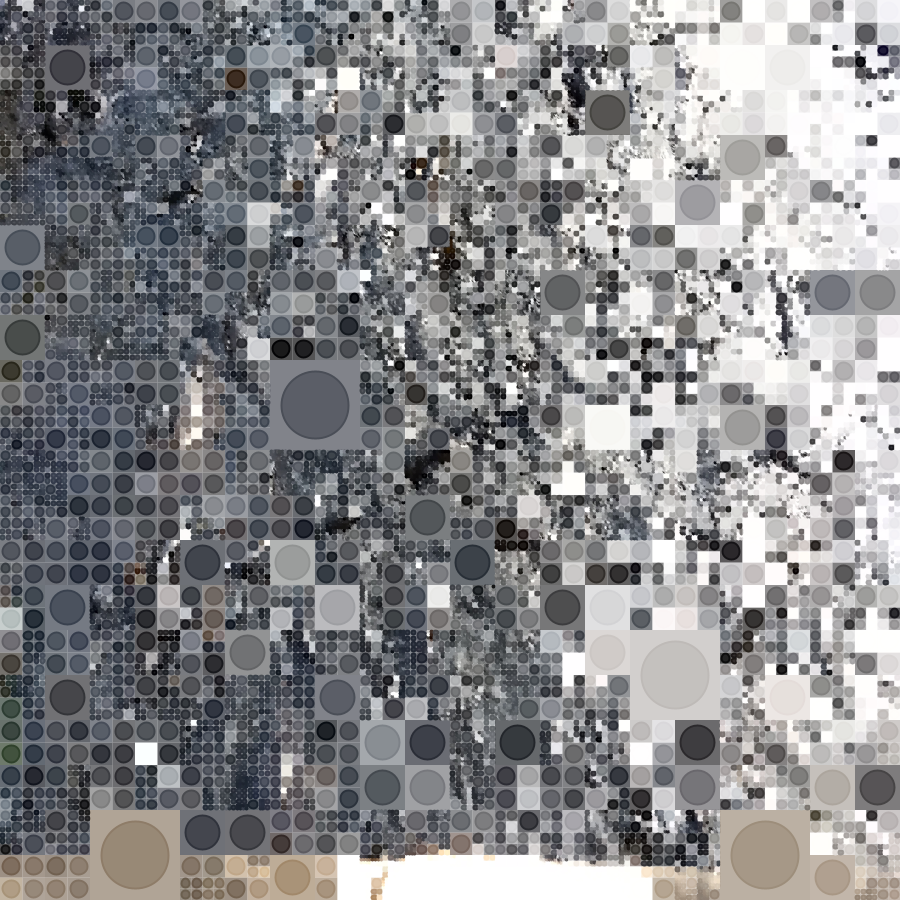### Approach 2 - Colors from an image extracted palette

Let’s work with the sunset image again:

Here’s the image:And here’s the code for creating a palette from the frequently used colors in the image:

``````val img = image("sunset-tree.png")
// step 1 - build up a map of pixel colors to counts
val pixels = HashMap.empty[Color, Int]
repeatFor(0 until img.getHeight) { y =>
repeatFor(0 until img.getWidth) { x =>
val p = getImagePixel(img, x, y)
pixels(p) = pixels.getOrElseUpdate(p, 0) + 1
}
}
// step 2 - create the palette from the map
val palette = pixels.toSeq.sortWith(_._2 > _._2).map(_._1).drop(2000).take(50)

def cellColor = randomFrom(palette)
``````

In the first step above, a map is created by going through all the pixels in the image, getting the color of each pixel, and putting these colors in a map along with their counts. Each time a previously seen color is encountered, it’s count is bumped up by 1.

In the second step (which is just one line of code!), many things happen:

• The map is converted to a sequence of (Color, Int) tuples (`pixels.toSeq`). Read more about tuples.
• This sequence is sorted in descending order based on the second elements (which are the color counts) of the tuples inside it (`.sortWith(_._2 > _._2)`).
• The sequence is mapped to another sequence which contains just the colors and not the counts, which we don’t need any more after sorting (`.map(_._1)`).
• The first 2000 elements of the new sequence are dropped, to get to some interesting colors (`.drop(2000)`)).
• The next 50 elements of the new sequence are put into the palette (`.take(50)`).

Here’s the full code and output:

``````size(900, 900)
cleari()
setBackground(white)
originBottomLeft()
initRandomGenerator(-647255116900691681L)

val tileCount = 3
val tileWidth = cwidth / tileCount
val tileHeight = cheight / tileCount

val img = image("sunset-tree.png")
val pixels = HashMap.empty[Color, Int]
repeatFor(0 until img.getHeight) { y =>
repeatFor(0 until img.getWidth) { x =>
val p = getImagePixel(img, x, y)
pixels(p) = pixels.getOrElseUpdate(p, 0) + 1
}
}
val palette = pixels.toSeq.sortWith(_._2 > _._2).map(_._1).drop(2000).take(50)

def cellColor = randomFrom(palette)

def shape(w: Double, h: Double) = {
val len = math.min(w, h) * 3 / 4
picStackCentered(noPen -> Picture.rectangle(w, h), Picture.ellipseInRect(len, len))
}

case class Block(x: Double, y: Double, w: Double, h: Double, c: Color)
var blocks = ArrayBuffer.empty[Block]

def drawBlock(b: Block) {
val pic = shape(b.w, b.h)
pic.setPosition(b.x, b.y)
val d = mathx.distance(b.x, b.y, mouseX, mouseY)
val f = mathx.map(d, 0, 2000, 0.3, .9)
val angle = mathx.angle(b.x, b.y, mouseX, mouseY)
pic.setPenColor(b.c)
pic.setPenThickness(2)
draw(pic)
}

def splitSomeBlocks(blocks: ArrayBuffer[Block], p: Double): ArrayBuffer[Block] = {
val blocks2 = ArrayBuffer.empty[Block]
repeatFor(blocks) { b =>
if (randomDouble(1) < p) {
val newBlocks = Array(
Block(b.x, b.y, b.w / 2, b.h / 2, cellColor),
Block(b.x, b.y + b.h / 2, b.w / 2, b.h / 2, cellColor),
Block(b.x + b.w / 2, b.y, b.w / 2, b.h / 2, cellColor),
Block(b.x + b.w / 2, b.y + b.h / 2, b.w / 2, b.h / 2, cellColor)
)
blocks2.appendAll(newBlocks)
}
else {
blocks2.append(b)
}
}
blocks2
}

setup {
repeatFor(rangeTill(0, cheight, tileHeight)) { posY =>
repeatFor(rangeTill(0, cwidth, tileWidth)) { posX =>
val block = Block(posX, posY, tileWidth, tileHeight, cellColor)
blocks.append(block)
}
}
repeat(5) {
blocks = splitSomeBlocks(blocks, 0.4)
}
}

drawLoop {
erasePictures()
repeatFor(blocks) { b =>
drawBlock(b)
}
}
``````